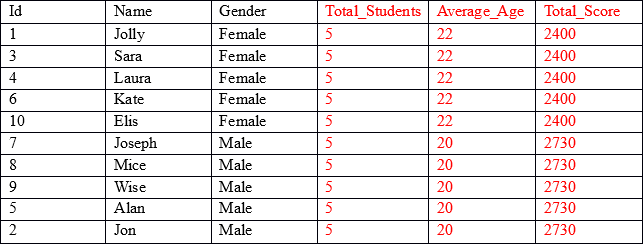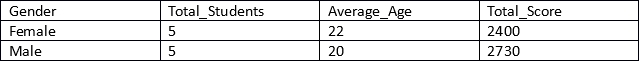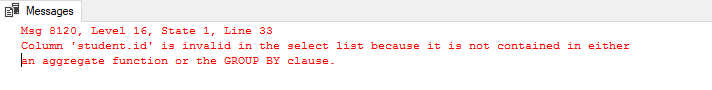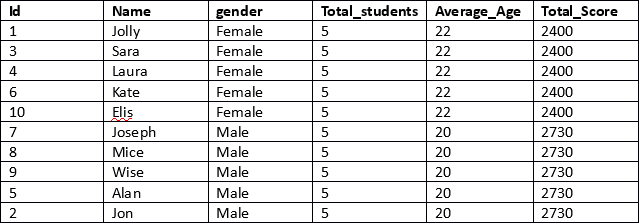15:40 • One Comment

# Grouping Data using the OVER and PARTITION BY FunctionsThe OVER and PARTITION BY functions are both functions used to portion a results set according to specified criteria.

This article explains how these two functions can be used in conjunction to retrieve partitioned data in very specific ways.

## Preparing Some Sample Data

To execute our sample queries, let’s first create a database named “studentdb”.

Run the following command in your query window:

`CREATE DATABASE schooldb;`

Next, we need to create the “student” table within the “studentdb” database. The student table will have five columns: id, name, age, gender, and total_score.

As always, make sure you are well backed up before experimenting with a new code. See this article on backing up SQL Server databases if you’re not sure.

Execute the following query to create the student table.

```USE schooldb
CREATE TABLE student
(
id INT PRIMARY KEY IDENTITY,
name VARCHAR(50) NOT NULL,
gender VARCHAR(50) NOT NULL,
age INT NOT NULL,
total_score INT NOT NULL,
)```

Finally, we need to insert some dummy data for us to work with into the database.

```USE schooldb
INSERT INTO student

VALUES ('Jolly', 'Female', 20, 500),
('Jon', 'Male', 22, 545),
('Sara', 'Female', 25, 600),
('Laura', 'Female', 18, 400),
('Alan', 'Male', 20, 500),
('Kate', 'Female', 22, 500),
('Joseph', 'Male', 18, 643),
('Mice', 'Male', 23, 543),
('Wise', 'Male', 21, 499),
('Elis', 'Female', 27, 400);```

Right we’re now ready to work on a problem and see who we can use Over and Partition By to solve it.

## Problem

We have 10 records in the student table and we want to display the name, id, and gender for all of the students, and in addition we also want to display the total number of students that belong to each gender, the average age of the students of each gender and the sum of the values in the total_score column for each gender.

The result set that we’re looking for is as below:As you can see, the first three columns (shown in black) contain individual values for each record, while the last three columns (shown in red) contain aggregated values grouped by the gender column. For example, in the Average_Age column, the first five rows display the average age and the total score of all the records where gender is Female.

Our result set contains aggregated results joined with non-aggregated columns.

To retrieve the aggregated results, grouped by a particular column, we can use the GROUP BY clause as usual.

```USE schooldb
SELECT gender, count(gender) AS Total_Students, AVG(age) as Average_Age, SUM(total_score) as Total_Score
FROM student
GROUP BY gender```

Let’s see how we can retrieve Total_Students, Average_Age, and Total_Score of the students grouped by gender.

You will see following results:Now let’s extend this and add ‘id’ and ‘name’ (the non-aggregated columns in the SELECT statement) and see if we can get our desired result.

```USE schooldb
SELECT id, name, gender, count(gender) AS total_students, AVG(age) as Average_Age, SUM(total_score) as Total_Score
FROM student
GROUP BY gender```

When you run the above query, you will see an error:The error says that the id column of the student table is invalid within the SELECT statement since we are using GROUP BY clause in the query.

This means that we will have to apply an aggregate function on the id column or we will have to use it in the GROUP BY clause. In short, this scheme doesn’t solve our problem.

## Solution Using JOIN Statement

One solution to this would be to make use of the JOIN statement to join the columns with aggregated results to columns containing non-aggregated results.

To do so, you need a sub-query that retrieves gender, Total_Students, Average_Age and the Total_Score of the students grouped by gender. These results can then be joined to the results obtained from the sub-query with the outer SELECT statement. This will be applied to the gender column of the sub-query containing the aggregated result and the gender column of the student table. The outer SELECT statement would include non-aggregated columns i.e. ‘id’ and ‘name’, as below.

```USE schooldb
SELECT id, name, Aggregation.gender, Aggregation.Total_students, Aggregation.Average_Age, Aggregation.Total_Score
FROM student
INNER JOIN
(SELECT gender, count(gender) AS Total_students, AVG(age) AS Average_Age, SUM(total_score) AS Total_Score
FROM student
GROUP BY gender) AS Aggregation
on Aggregation.gender = student.gender```

The above query will give you the desired result but is not the optimal solution. We had to use a JOIN statement and a sub-query which increases the complexity of the script. This is not an elegant or efficient solution.

A better approach is to use the OVER and PARTITION BY clauses in conjunction.

## Solution Using OVER and PARTITION BY

To use the OVER and PARTITION BY clauses, you simply need to specify the column that you want to partition your aggregated results by. This is best explained with the use of an example.

Let’s have a look at achieving our result using OVER and PARTITION BY.

```USE schooldb
SELECT id, name, gender,
COUNT(gender) OVER (PARTITION BY gender) AS Total_students,
AVG(age) OVER (PARTITION BY gender) AS Average_Age,
SUM(total_score) OVER (PARTITION BY gender) AS Total_Score
FROM student```

This is a much more efficient result. In the first line of the script the id, name and gender columns are retrieved. These columns do not contain any aggregated results.

Next, for the columns that contain aggregated results, we simply specify the aggregated function, followed by the OVER clause and then within the parenthesis we specify the PARTITION BY clause followed by the name of the column that we want our results to be partitioned as shown below.